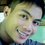# The Faster Manual Method of Finding the Center and the Radius of the Circle's General Equation

Do you have difficulty in finding the center and the radius of the general form of a circle's equation? If yes, then relax. 🐛🐝

Be cool!🍨🍦🍧🍹

Grouping of terms with variables x and y ,and completing the squares are not needed using these shortcuts.

Are you ready to learn? ☺📚📖☺

We usually look for the faster manual way or method on how to find the center and the radius of the general form of circle's equation, don't we?

The general form of the circle's equation is x²+y²+ax+by+c=0.👍

Here is an example. 👇

Sample Problem:

What is the center and the radius of

x²+y²-2x-12y-63=0 ? 📚⏳

Given: a= -2, b= -12, and c= -63

Solution:

Shortcut in finding the coordinates of the circle's center

C(h,k)= (a/-2, b/-2) 👈FORMULA👨

Substitute -2 for a and -12 for b.

C(h,k)= (-2/-2),-12/-2)

C(h,k)= (1,6) ✔👍 center

Shortcut in finding the circle's radius length

Let sqrt stands for the square root.

r = sqrt(h²+k² -c) 👈FORMULA👨

Substitute 1 for h, 6 for k, and -63 for c.

r = sqrt(1² +6² -(-63))

r = sqrt(1+ 36 +63)

r = sqrt(100)

r = 10 units ✔👍 radius length

Hence, the circle's center is at point C(1,6) and its radius length 10 units.

Now, you try! ☺

Exercises: 📚

Solve the center and the radius length of each circle's equation.

1. x²+y²-4x+8y-9=0

2. x²+y²+10x-18y+11=0

3. x²+y²+2y-3=0

⏰


You can also calculate each circle's diameter, circumference and area given its computed radius length.

Author: John Paul L. Hablado, LPT4 years, 3 months ago

This discussion board is a place to discuss our Daily Challenges and the math and science related to those challenges. Explanations are more than just a solution — they should explain the steps and thinking strategies that you used to obtain the solution. Comments should further the discussion of math and science.

When posting on Brilliant:

• Use the emojis to react to an explanation, whether you're congratulating a job well done , or just really confused .
• Ask specific questions about the challenge or the steps in somebody's explanation. Well-posed questions can add a lot to the discussion, but posting "I don't understand!" doesn't help anyone.
• Try to contribute something new to the discussion, whether it is an extension, generalization or other idea related to the challenge.

MarkdownAppears as
*italics* or _italics_ italics
**bold** or __bold__ bold
- bulleted- list
• bulleted
• list
1. numbered2. list
1. numbered
2. list
Note: you must add a full line of space before and after lists for them to show up correctly
paragraph 1paragraph 2

paragraph 1

paragraph 2

[example link](https://brilliant.org)example link
> This is a quote
This is a quote
    # I indented these lines
# 4 spaces, and now they show
# up as a code block.

print "hello world"
# I indented these lines
# 4 spaces, and now they show
# up as a code block.

print "hello world"
MathAppears as
Remember to wrap math in $$ ... $$ or $ ... $ to ensure proper formatting.
2 \times 3 $2 \times 3$
2^{34} $2^{34}$
a_{i-1} $a_{i-1}$
\frac{2}{3} $\frac{2}{3}$
\sqrt{2} $\sqrt{2}$
\sum_{i=1}^3 $\sum_{i=1}^3$
\sin \theta $\sin \theta$
\boxed{123} $\boxed{123}$

Sort by: